# Mental Division of Large Numbers

Mental Division of Large Numbers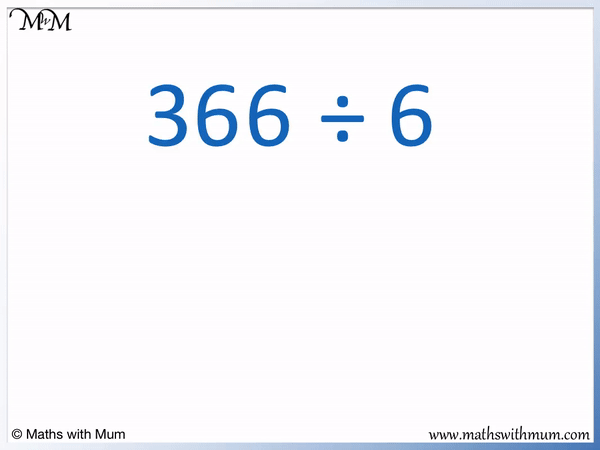• If the digits of a number can be grouped into multiples of the number being divided by, we can divide these groups separately.
• 366 can be partitioned into 36 tens and 6.
• We can divide 36 tens and 6 both by 6 exactly.
• 36 ÷ 6 = 6 and so, there are 6 tens in the answer.
• 6 ÷ 6 = 1 and so, there is 1 unit in the answer.
• 6 tens and 1 unit add to make 61.
• 366 ÷ 6 = 61.
• We try to find groups of digits that can be divided exactly.
• If this cannot be done, there will be a remainder in the division.
Group the digits into numbers that can be divided exactly.• 729 can be partitioned into 72 tens and 9 ones.
• We can divide 72 tens and 9 separately to find the answer.
• 72 ÷ 9 = 8, therefore there are 8 tens in the answer.
• 9 ÷ 9 = 1 and so, there is 1 unit in the answer.
• 729 ÷ 9 = 81.Supporting Lessons# Mental Division of Larger Numbers

## How to Divide a Number by Partitioning

To divide a number by partitioning, write the number as the sum of two or more smaller numbers that can be divided exactly. Divide these numbers and then add the results to find the answer.

Partitioning simply means to write a number as two or more smaller numbers added together.

For example in 44 ÷ 2, 44 can be written as 40 + 4.

We choose to partition 44 into 40 and 4 because both 40 and 4 can be divided easily by 2.4 ÷ 2 = 2 and so, 40 ÷ 2 = 20.

We can simply divide the digits one at a time.

44 ÷ 2 = 22

Both digits of 44 can be simply divided by 2 to make the digits of the answer.

A number can be easily divided mentally if the digits can be grouped into numbers that divide exactly.

For example, here is 63 ÷ 3. We can see that both digits 6 and 3 can be divided by 3.

6 ÷ 3 = 2 and so, the tens digit of the answer is 2.3 ÷ 3 = 1 and so, the ones digit of the answer is 1.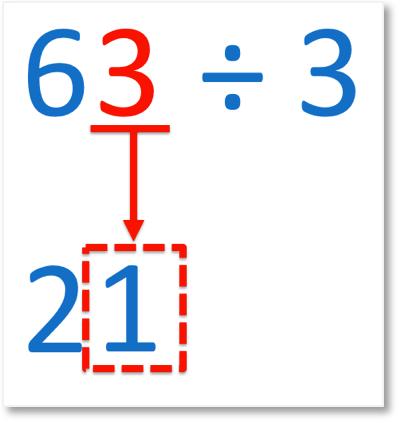The digits in the number can be divided exactly and so, this division can be done mentally.If all digits of a number can be divided exactly then the division can be done by dividing each digit individually. If this is not possible, it may be possible to group the digits into numbers that are divisible exactly.

For example, here is 128 ÷ 2.

Looking at the first digit, 1 is not divisible by 2 exactly. If the first digit of the number cannot be divided exactly, combine it with the next digit and see if this new number is divisible exactly.

The first two digits of 128 is 12. 12 can be divided by 2.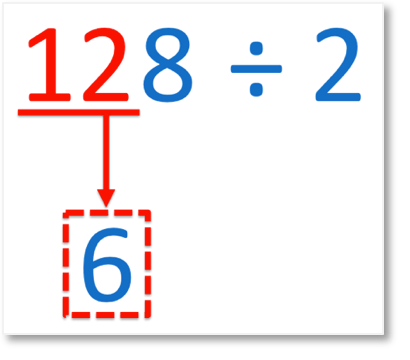12 ÷ 2 = 6 and so, there are 6 tens in the answer.

Now all that remains is to divide the 8 by 2.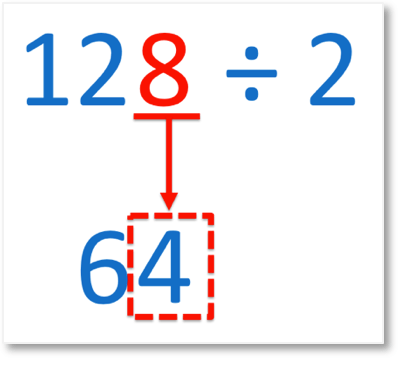8 ÷ 2 = 4 and so, there are 4 ones in the answer.

The final step is to combine the digits of the answer. 128 ÷ 2 = 64.

Here is another example of 105 ÷ 5.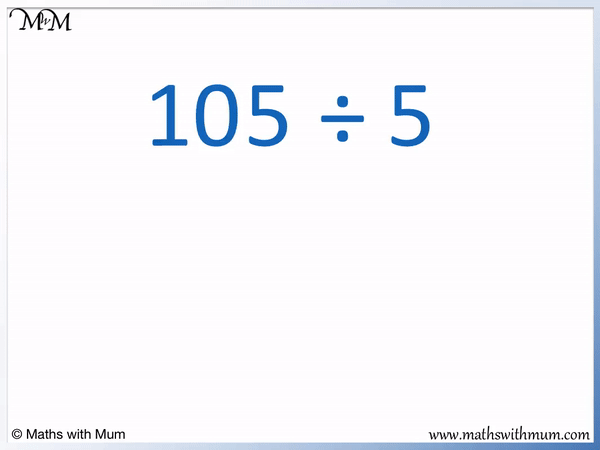1 is not divisible by 5 and so, we combine it with the next digit along to make 10.

10 ÷ 5 = 2, therefore there are 2 tens in the answer.

The remaining digit is 5. 5 ÷ 5 = 1 and so, there is 1 unit in the answer.

105 ÷ 5 = 21

## The Split Strategy for Division

The split strategy for division is a method used to divide larger numbers by writing them as the sum of smaller numbers. Divide the smaller numbers first and then add the results to find the answer.

The split strategy for division is another name for division by partitioning. When teaching these methods, it can be helpful to start by partitioning the numbers into tens and ones.

For example, 86 ÷ 2 can be written as 80 + 6.

Since 8 ÷ 2 = 4, 80 ÷ 2 = 40.

6 ÷ 2 = 3.

>40 + 3 = 43 and so, 86 ÷ 2 = 43.

It does not matter how the number is split when using the split strategy. For example, we could write 86 as 60 + 26.

We then divide these two numbers. 60 ÷ 2 = 30 and 26 ÷ 2 = 13.

We add the results so that 30 + 13 = 43. We still get the same answer.

Here is another example of 729 ÷ 9.We can split 729 into 720 + 9.

72 ÷ 9 = 8 and so, 720 ÷ 9 = 80.

9 ÷ 9 = 1.

80 + 1 = 81 and so, 729 ÷ 9 = 81.

Again we could have split 729 into any other combination of numbers as long as they are divisible by 9.

For example, 729 can be written as 630 + 99.

630 ÷ 9 = 70 and 99 ÷ 9 = 11.

70 + 11 = 81 and so, the answer is the same.

Division by partitioning or the split strategy, teaches students to break down larger numbers into manageable chunks. These methods are used to build up strategies for mental division.

If a number cannot be divided exactly, then there will be a remainder or the division answer will be a decimal.

For example, if we have 206 ÷ 5, we can see that 200 ÷ 5 = 4, however 6 ÷ 5 = 1 remainder 1.

206 ÷ 5 = 41 remainder 1.Now try our lesson on Short Division without Remainders where we introduce the short division method.error: Content is protected !!